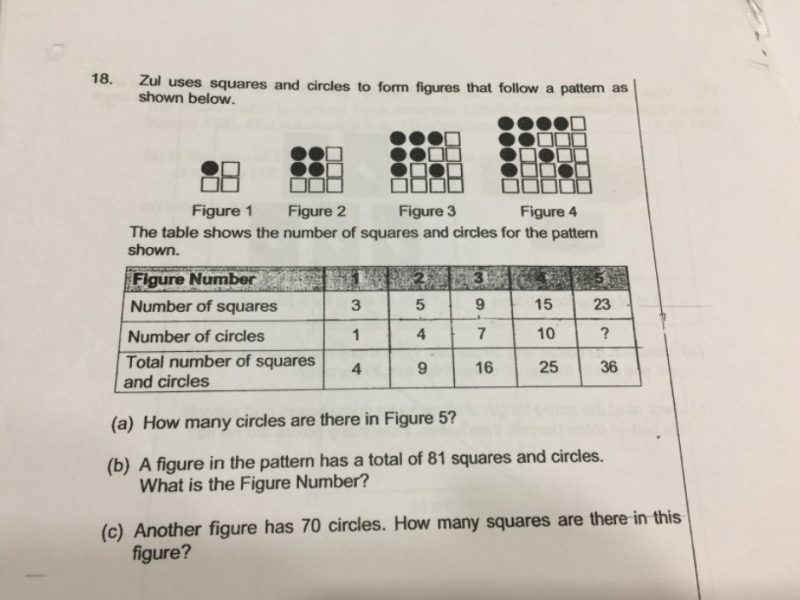# QuestionLet n be the figure number.

Then the total number of squares and circles = (n + 1)²

For the number of circles, observe that the difference is 3.

The number of circles is 3n – 2.

The number of squares is obtained by subtracting the number of circles from the total number of squares and circles.

(a) This can be worked out easily.

(b) (n + 1)² = 81, so n = 8. It is Figure Number 8.

(c) 3n – 2 = 70 so n = 24.

Total number of squares and circles = (24 + 1)² = 25² = 625

The number of squares = 625 – 70 = 555

0 Replies 0 Likes ✔Accepted Answer